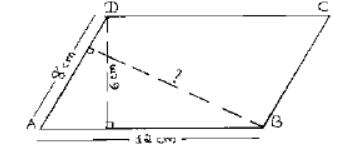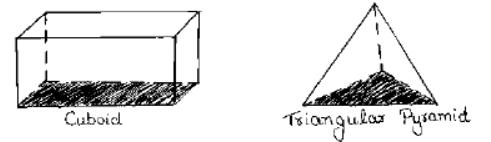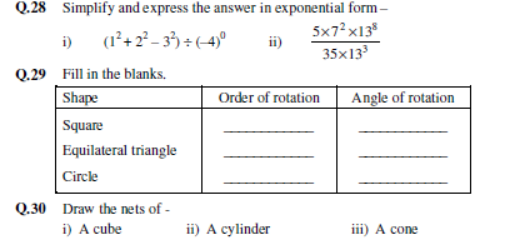# CBSE Class 7 Mathematics Sample Paper Set O

Read and download PDF of CBSE Class 7 Mathematics Sample Paper Set O designed as per the latest curriculum and examination pattern for Class 7 issued by CBSE, NCERT and KVS. The latest Class 7 Mathematics Sample Papers have been provided with solutions so that the students can solve these practice papers and then compare their answers. This will help them to identify mistakes and improvement areas in Mathematics Standard 7 which they need to study more to get better marks in Grade 7 exams. After solving these guess papers also refer to solved Class 7 Mathematics Question Papers available on our website to build strong understanding of the subject

## Sample Paper for Class 7 Mathematics Pdf

Students can refer to the below Class 7 Mathematics Sample Paper designed to help students understand the pattern of questions that will be asked in Grade 7 exams. Please download CBSE Class 7 Mathematics Sample Paper Set O

### Mathematics Class 7 Sample Paper

CBSE Class 7 Mathematics Sample Paper Set O. It’s always recommended to practice as many sample papers as possible before the examinations. Students can download the sample papers and also question papers of previous years to practice and score better marks in examinations. Refer to other links too for more sample papers.

SECTION-A

Q.1 Find the ratio of 30 minutes to 1 hour.

Q.2 What is the coefficient of y2 in –2x2y2 ?

Q.3 Write 6389014 in expanded form.

Q.4 What other name can you give to the line of symmetry of a circle?

Q.5 What is the sum of numbers on opposite faces of a dice?

SECTION - B

Q.6 If Rs. 250 is to be divided amongst Kavya, Shruti and Sneha in the ratio 2:3:5, how much money will each get?

Q.7 Write 3 rational numbers between 1/3 and 2/5.

Q.8 The perimeter of a rectangular sheet is 120 cm. If its length is 40 cm, find the breadth. Also find its area.

Q.9 Simplify the following expression and find its value when x = 7 and y = –2.

2(x2+xy) + 7 – xy.

Q.10 Compare 2.7×1012 and 1.5×108.

SECTION-C

(Attempt any 10 questions)

Q.11 During a sale, the price of a toy is reduced from Rs. 800 to Rs. 600. Find the percentage decrease in the price.

Q.12 If Harshit pays an interest of Rs. 600 for 5 years on a sum of Rs. 3000, find the rate of interest.

Q.13 Arrange the rational numbers 5/-6 , -7/9 , 0, 2/-3 in ascending order.

Q.14 Draw a line PQ. Take a point R outside it. Through R, draw a line parallel to PQ using ruler and compass.

Q.15 Construct a ΔXYZ with XY=6 cm, YZ = 7 cm and ZX = 5.5 cm.

Q.16 Two sides of a parallelogram are 12 cm and 8 cm. If the altitude corresponding to the side of length 12 cm is 6 cm long, find the length of altitude corresponding to side 8 cm.Q.17 If the circumference of a circular sheet is 154 m, find the area of the sheet.

Q.18 Frame the algebraic expression for the following :

i) Two times a number n increased by six.

ii) A number p multiplied by itself.

iii) Subtraction of p from q.

iv) Product of numbers y and z subtracted from 20.

v) Half of a number m added to 7.

Q.19 i) Express 64 × 243 as a product of prime factors.

ii) Write 6894321 in standard form.

Q.20 What letters of the English alphabet have reflectional symmetry about –

i) a vertical mirror

ii) a horizontal mirror

iii) both horizontal and vertical mirrors.

Q.21 State the number of lines of symmetry for the following figures –

i) an equilateral triangle ii) An isosceles triangle iii) A scalene triangle

iv) a square v) a rectangle vi) a circle

Q.22 Write the number of faces, edges and vertices of the following shapes.SECTION-D

(Attempt any 5 questions)

Q.23 i) Chalk contains calcium, carbon and oxygen in the ratio 10:3:12. Find the percentage of carbon in chalk.

ii) Rahul bought a toy car for Rs. 1000. His friend Sahil liked the toy car and wanted to have

it for himself, but could not afford to buy it. So Rahul sold the toy car to Sahil for Rs. 500.

a) Calculate the loss percent that Rahul had.

b) What value is depicted by Rahul through the above action?Q.25 Construct ΔPQR if PQ=5 cm, ∠PQR = 1050 and ∠QRP = 400.

Q.26 Two crossroads, each of width 3 m, run at right angles through the centre of a rectangular park of length 80 m and breadth 70 m and parallel to its sides. Find the area of the roads. Also find the area of the park excluding crossroads.

Q.27 Subtract y2 – 3y – 4 from the sum of 4y2 – 2y + 3, –3y2 + 6 and –5y2 + 6y.